# Circle TheoremsPage 1

#### WATCH ALL SLIDES

Slide 1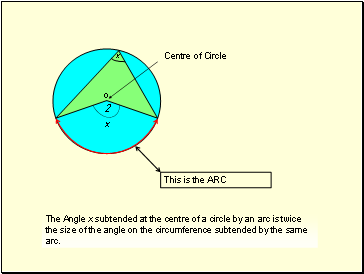The Angle x subtended at the centre of a circle by an arc is twice the size of the angle on the circumference subtended by the same arc.

Slide 2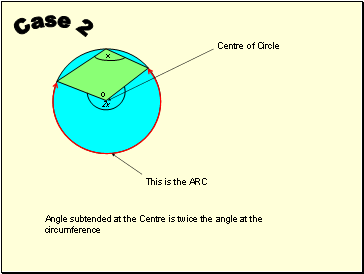## Case 2

2x

x

o

Angle subtended at the Centre is twice the angle at the circumference

Slide 3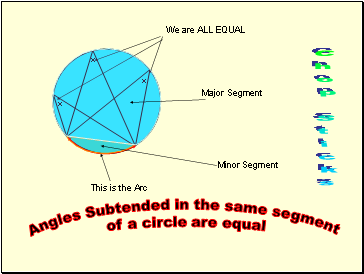## Chop Sticks

Angles Subtended in the same segment

of a circle are equal

Slide 4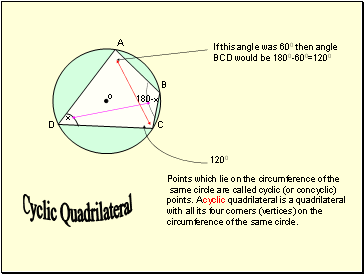A

B

C

D

Points which lie on the circumference of the

same circle are called cyclic (or concyclic)

with all its four corners (vertices) on the

circumference of the same circle.

Slide 5## Tangents

T

A

B

O

TA=TB

NB Triangles

OBT and OAT

are CONGRUENT!

Slide 6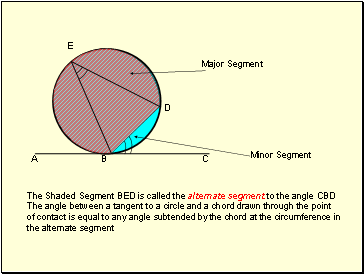A

B

C

E

D

The Shaded Segment BED is called the alternate segment to the angle CBD

The angle between a tangent to a circle and a chord drawn through the point

of contact is equal to any angle subtended by the chord at the circumference in

the alternate segment

Slide 7## Diameter

The angle in a semi circle is 90 degrees!